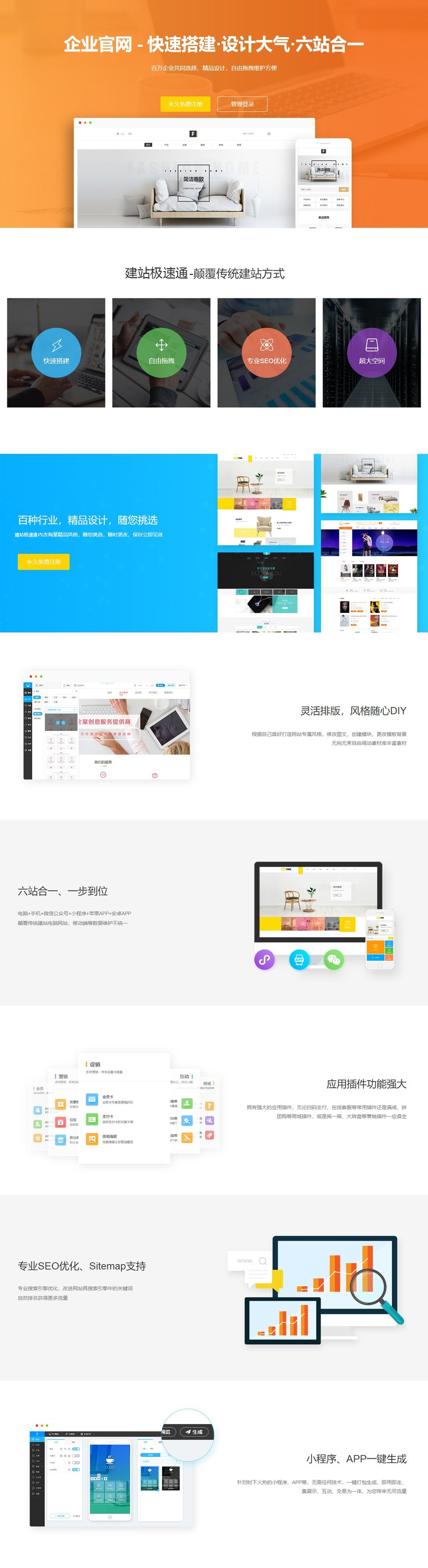### (独享服务器+HTTPS+AI名片+一站千词)# 企业官网￥1480
 累计销量1732 累计评价0

1.创业扶持
2.专属客服
3.稳定运行
4.自助建站•• 服务项目
• 功能对比
• 所有评价展示免费版 展示入门版 展示标准版 展示营销版 展示尊享版 （免费） （360） （680） （980） （1480） 展示型网站版本及功能说明 版本信息 首次购买送初建服务 X X X √ √ 首次购买送备案服务 X X X √ √ 百度不收录,全额退款 (30天后不收录,全额退款) X X X √ √ 隐藏技术支持 X X √ √ √ 首次购买送1年独立域名 X √ √ √ √ 首次购买送企业邮箱券 X √ √ √ √ 首次购买送400号码券 X √ √ √ √ 去广告块 X √ √ √ √ 网站空间 网站空间 500M 5G 10G 100G 2T 文件数量 100个 1万个 10万个 20万个 不限 单个文件大小 10M 20M 30M 40M 50M 基本功能 文章批量上传 X √ √ √ √ 产品搬家 X √ √ √ √ 404页面设计 X √ √ √ √ 文章数量 100篇 5000篇 1万篇 2万篇 10万篇 产品数量 100个 5000个 1万个 2万个 10万个 图册数量 10个 1000个 5000个 1万个 2万个 网站栏目 20个 100个 200个 500个 1000个 手机空白页 10个 100个 200个 500个 1000个 自定义模块数量 500个 1000个 2000个 5000个 1万个 会员数量 100个 1万个 2万个 5万个 10万个 互动功能 访客留言数量 100条 1万条 2万条 5万条 不限 在线表单数量 10个 100个 200个 500个 1万个 表单提交数量 100条 1万条 2万条 5万条 不限 会员功能 会员积分 X X X X √ 浏览权限 X X X √ √ 会员信息 X X X √ √ 会员等级 X X X √ √ 会员发布 X X X √ √ 邀请好友 X X X √ √ 第三方登录 X X √ √ √ 会员公告 X X √ √ √ 会员概要 √ √ √ √ √ 会员管理 √ √ √ √ √ 会员设置 √ √ √ √ √ 营销插件 论坛 X X X X √ 微签到 X X X √ √ 微投票 X X X √ √ 微报名 X X X √ √ 微调研 X X X √ √ 邀请函 X X X √ √ 站内广告 X X X √ √ 微海报 X X X √ √ 下载功能 X X X √ √ 万能查询 X X X √ √ 微页 X X X √ √ 背景音乐 X √ √ √ √ 表单系统 √ √ √ √ √ 在线客服 √ √ √ √ √ 用户留言 √ √ √ √ √ 欢迎页 √ √ √ √ √ 用户评论 √ √ √ √ √ 互动游戏 全民抢红包 X X X √ √ 拾金蛋 X X X √ √ 摇一摇 X X X √ √ 砸金蛋 X X X √ √ 翻牌游戏 X X X √ √ 水果机 X X X √ √ 刮刮卡 X X X √ √ 大转盘 X X X √ √ 搜索引擎优化 网站关键字和描述 X √ √ √ √ 网站html静态化 X √ √ √ √ 网站sitemap X √ √ √ √ 301跳转 X √ √ √ √ 公众号 群发 X X X √ √ 微信账户 X √ √ √ √ 微信菜单 X √ √ √ √ 素材库 X √ √ √ √ 关注回复语 X √ √ √ √ 关键词应答 X √ √ √ √ 微信客服 X √ √ √ √ 粉丝 X √ √ √ √ 微信提醒 X √ √ √ √ 验证文件 X √ √ √ √ 小程序 微信小程序 × 按需购买 按需购买 按需购买 按需购买 百度小程序 APP 一键生成安卓App × 按需购买 按需购买 按需购买 按需购买 一键生成苹果App 熊掌号 熊掌号对接 X X X √ √ 扩展支持 消息提醒 X X X √ √ 网站验证 X √ √ √ √ 网站小图标 X √ √ √ √ 网站安全 X √ √ √ √ 嵌入javascript/css模块 X √ √ √ √ 嵌入第三方页面 X √ √ √ √ 嵌入第三方统计工具 X √ √ √ √ 安全与服务 https安全 X X X √ √ 短信消息提醒 X √ √ √ √ 数据备份（按天） X √ √ √ √ 24小时监控服务 X √ √ √ √ 双线接入 X √ √ √ √ CDN网络加速 X √ √ √ √ 邮件即时提醒 X √ √ √ √ 导出数据 X √ √ √ √ 员工操作日志 X √ √ √ √ 建站咨询服务 √ √ √ √ √ 保存历史记录 √ √ √ √ √
 与描述相符 0 非常不满 不满意 一般 满意 非常满意## 客服QQ：## 建站学院• ## 电话直呼

• 0871-68506514
• 售前客服 ：
• 售前客服 ：
• 设计支持 ：
• 售后客服 ：
• 售后客服 ：
• 技术支持 ：
• 代理加盟 ：
•扫一扫关注我们×